﻿ Predicting Oil Production Level for Specified Cost, Price and Revenue

### Predicting Oil Production Level for Specified Cost, Price and Revenue

C. I. Nwoye, I. D. Adiele, S. E. Ede, I. H. Obiagwu, C. C. NwoyeOPEN ACCESSPEER-REVIEWED

## Predicting Oil Production Level for Specified Cost, Price and Revenue

C. I. Nwoye1,, I. D. Adiele2, S. E. Ede3, I. H. Obiagwu4, C. C. Nwoye5

1Department of Metallurgical and Materials Engineering, Nnamdi Azikiwe University, Awka, Nigeria

2Department of Engineering Research Development and Production, Project Development Institute, Enugu, Nigeria

3Department of Metallurgical and Materials Engineering, Enugu State University of Science & Technology, Enugu, Nigeria

4United Bank for Africa, Lagos, Nigeria

5Robert Gordon University, Aberdeen

### Abstract

This paper presents an evaluation of the trend of oil level production dependence on its operating cost, current oil price and expected revenue over a production period of 10 years (2003-2012). Results from both experiment and model prediction show that the oil production level is very significantly affected by its operating cost and expected revenue at a particular current oil price. A three-factorial model was derived, validated and used for the response analysis. Results from evaluations indicated that the standard error incurred in predicting oil production level for each value of the expected revenue & operating cost considered, as obtained from derived model and regression model were 0.0401 and 3.5 x 10-4 & 3.7348 and 3.7544% respectively. Further evaluation indicates that oil production per unit operating cost as obtained from experiment; derived model and regression model were 0.0556, 0.0552 and 0.0552 MTSB /M\$ respectively. Comparative analysis of the correlations between oil production and operating cost, current oil price and expected revenue as obtained from experiment; derived model and regression model indicated that they were all > 0.99. The maximum deviation of the model-predicted oil production level (from experimental results) was less than 7%. This translated into over 93% operational confidence for the derived model as well as over 0.93 reliability response coefficients of oil production dependence to the operating cost, current oil price and expected revenue.

### At a glance: Figures

123456
Prev Next

• Nwoye, C. I., et al. "Predicting Oil Production Level for Specified Cost, Price and Revenue." International Journal of Econometrics and Financial Management 2.6 (2014): 236-242.
• Nwoye, C. I. , Adiele, I. D. , Ede, S. E. , Obiagwu, I. H. , & Nwoye, C. C. (2014). Predicting Oil Production Level for Specified Cost, Price and Revenue. International Journal of Econometrics and Financial Management, 2(6), 236-242.
• Nwoye, C. I., I. D. Adiele, S. E. Ede, I. H. Obiagwu, and C. C. Nwoye. "Predicting Oil Production Level for Specified Cost, Price and Revenue." International Journal of Econometrics and Financial Management 2, no. 6 (2014): 236-242.

 Import into BibTeX Import into EndNote Import into RefMan Import into RefWorks

### 1. Introduction

The enormous monetary loss associating oil exploration and production as a result of storm damage to offshore installations and other vices has raised the need for evaluation of the inherent oil production risk and development of risk management system.

Research  has shown that different organizations approached risk analysis from various perspectives depending on the company’s policies. The researchers’ identified some problems as the cause of poor risk analysis procedures such as wrong concepts and miscommunication by the risk analysis staff. Report of the research identified the risk associated with investments in oil exploration and production as risk of damage to offshore installations; risk relating to future oil and gas prices; risk of exploration or development of dry hole and environmental risk.

Many researches [2, 3, 4, 5] have been hinged on risk and decision analysis, even though the inability to apply effectively the risk analysis tools has been one of the major set back 

Studies [2, 7] have shown the need to go through the process of screening which entails carrying out an economic feasibility study of an investment possibility and rate its investment opportunity. Report from these studies also posited ranking as a process of prioritizing accepted projects with respect to the available funds, corporate policy and objectives.

The oil exploration production is basically a capital intensive business that frequently makes use of economic analysis in assessing and evaluating the viability of projects that will consume investment capital.

Based on the foregoing, it is not economical to embark on field work always in order to generate data that would be useful in making future decisions in oil exploration and production. In other words, money and time could save and risk reduced if data generated from a particular field work could be empirical modeled and possibly simulated to capture future production expectations, providing the process conditions are maintained. Predictions with very insignificant deviation or risk could be made by simply substituting the known production evaluation parameters into the derived model.

The aim of this work is to predict oil production level for specified cost, price and revenue. A model would be derived, validated and used as a tool for predictive analysis of the oil production level. This work is expected to assist the Engineer in predicting (with insignificant risk) the likely oil production level that would give the expected revenue, for a particular operating cost and current oil price. This would be achieved by just substituting the values of the expected revenue, operating cost and current oil price into the model to be derived. For the purpose of this work, deviation of model-predicted oil production level (from the experimental values) would be assumed as the risk on using the derived model for predicting oil production level instead of embarking on field work to actually produce the oil and possibly evaluate the production level. Furthermore, field work would be assumed to be experimental work.

### 2. Methodology

2.1. Source of Data

Data on petroleum field development, capital costs, operating costs, field life and other data were collected from Devcon Exploration and Production Inc. (located around South Texas and South America) . These data were considered for Cuulon Project.

2.2. Model Formulation

#### Table 1. Variation of oil production level with current oil price, expected revenue and operating cost 

Computational analysis of the experimental data  shown in Table 1, gave rise to Table 2 which indicate that;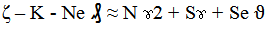(1)

Introducing the values of the empirical constants K, N, S, Se and Ne into equation (1) reduces it to;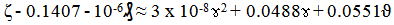(2)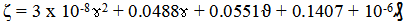(3)

Where

(ζ) = Oil production level (MSTB)

(ϑ) = Expected revenue (M\$)

(ɤ) = Current oil price (\$Bbl)

(₰) = Operating cost (M\$)

K = 0.1407, N = 3 x 10-8, S = 0.0488, Se = 0.0551 and ₰ = 10-6. These are empirical constant (determined using C-NIKBRAN .

### 3. Boundary and Initial Condition

The ranges of oil production level, expected revenue, current oil price and operating cost considered for the Cuulon Project are 1362-12918 MTSB, 24516-232524 M\$, 17-18 \$Bbl and 23666 – 231324 M\$ respectively. These data were

### 4. Results and Discussion

The derived model is equation (3). Results from both experiment and model prediction show that the oil production level is very significantly affected by its operating cost and expected revenue at a particular current oil price.

#### Table 2. Variation of ζ - 0.1407 - 10-6 ₰ with 3 x 10-8 ɤ2 + 0.0488ɤ + 0.0551ϑ

4.1. Model Validation

The validity of the model is strongly rooted on the core model expression: ζ - 0.1407 - 10-6₰ ≈ 3 x 10-8 ɤ2 + 0.0488ɤ + 0.0551ϑ where both sides of the expression are correspondingly approximately equal to 1. Table 2 also agrees with equation (2) following the values of ζ - 0.1407 - 10-6₰ and 3 x 10-8 ɤ2 + 0.0488ɤ + 0.0551ϑ evaluated from the experimental results in Table 1. Furthermore, the derived model was validated by comparing the model-predicted oil production level and that obtained from the experiment. This was done using the 4th Degree Model Validity Test Techniques (4th DMVTT); statistical graphical, computational and deviational analysis .

Figure 1. Variation of oil production level with expected revenue as obtained from experiment
4.2. Statistical Analysis
4.2.2. Correlation (CORREL)

The correlation coefficient between oil production level and expected revenue, current oil price and operating cost were evaluated (using Microsoft Excel Version 2003) from results of the experiment and derived model. These evaluations were based on the coefficients of determination R2 shown in Figure 1- Figure 6.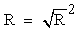(4)

The evaluated correlations are shown in Table 3- Table 5. These evaluated results indicate that the derived model predictions are significantly reliable and hence valid considering its proximate agreement with results from actual experiment.

#### Table 5. Comparison of the correlations evaluated from derived model predicted and experimental results based on operating cost

4.3. Graphical Analysis

Graphical analysis of Figure 7 and Figure 8 show very close alignment of curves and similar dimensions of covered areas. These designate oil production level from experimental (ExD) and model-predicted (MoD) results.

Figure 7. Comparison of oil production level (relative to expected revenue) as obtained from experiment and derived model

It is strongly believed that the degree of alignment of these curves is indicative of the proximate agreement between both experimental and model-predicted values of oil production.

4.3.1. Comparison of Derived Model with Standard Model

The validity of the derived model was further verified through application of the Least Square Method (LSM) in predicting the trend of the experimental results. Comparative analysis of Figure 10- Figure 12 shows very close alignment of curves and areas covered by oil production level, which precisely translated into significantly similar trend of data point’s distribution for experimental (ExD), derived model (MoD) and regression model-predicted (ReG) results of oil production level.

Also, the calculated correlations (from Figure 10- Figure 12) between oil production and expected revenue, current oil price and operating cost for results obtained from regression model gave 1.0000, 0.9622 and 1.0000 respectively. These values are in proximate agreement with both experimental and derived model-predicted results.

4.4. Computational Analysis

Computational analysis of the experimental, derived model and regression model-predicted oil production level was carried out to ascertain the degree of validity of the derived model. This was done by comparing the oil production level per unit operating cost obtained by calculation from experimental result and model-predictions.

4.4.1. Oil production per unit operating cost

The oil production per unit operating cost was calculated from the expression;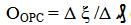(5)

Equation (5) is detailed as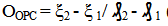(6)

Where

Δξ = Change in the magnitude of oil production at two operating costs ₰ 2, ₰ 1.

Considering the points (231324, 12918) & (23666, 1362), (231324, 12813.09) & (23666, 1351.851) and (231324,12802.73) & (23666, 1350.178) as shown in Figure 9, then designating them as (ζ1, ₰1) & (ζ2, ₰2) for experimental, derived model and regression model predicted results respectively, and also substituting them into equation (11), gives the slopes: 0.0556, 0.0552 and 0.0552 MTSB/ M\$ as their respective oil production per unit operating cost.

4.5. Deviational / Risk Analysis

Comparative analysis of the oil production level from experiment and derived model shows low deviations on the part of the model-predicted values relative to values obtained from the experiment. These deviations are regarded as risk when making predictions on Cuulon Project using the derived model. These deviations were attributed to the fact that the actual process conditions which prevailed and played vital roles during the production process were not considered during the model formulation. This necessitated the introduction of correction factor, to bring the model-predicted oil production level to those of the corresponding experimental values.

The deviation Dv, of model-predicted oil production level from the corresponding experimental result was given by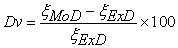(7)

Where

ξExD and ξMoD are oil production levels obtained from experiment and model-predicted results respectively.

Deviational/risk analysis from Figure 13 and Figure 14 indicates that the precise maximum deviation of model-predicted oil production level from the experimental results is 6.31%. This invariably translated into over 93% operational confidence for the derived model as well as over 0.93 reliability response coefficients of oil production dependence to the operating cost, current oil price and expected revenue.

Figure 13. Variation of model-predicted oil production level (based on production year) with associated deviation/ risk from experiment
Figure 14. Variation of model-predicted oil production level (relative to expected revenue) with associated deviation/ risk from experiment

Consideration of equation (9) and critical analysis of Figure 13 and Figure 14 shows that the least and highest magnitudes of deviation/ risk of the model-predicted oil (from the corresponding experimental values) are – 0.75 and – 6.31%. Figure 2, Figure 4, Figure 6, Figure 13 and Figure 14 indicate that these deviations correspond to oil production levels: 1351.851 and 2103.366 MTSB, operating cost: 23666 and 37306 M\$, expected revenue: 24516 and 38156 M\$ as well as current oil price: 18 and 17 \$Bbl respectively.

Figure 15. Variation of model-predicted oil production level (relative to current oil price) with associated deviation/ risk from experiment

4.5.1. Predictability of Oil Production Level using Derived Model

Comparative analysis of the oil production level from experiment and derived model (as shown in Figure 7, Figure 8 and Figure 13- Figure 15 clearly indicate that the derived model predicted (with insignificant risk) oil productivity levels that would realize the expected revenue (same realized from the actual experiment (field work)) for a particular operating cost and current oil price. This was achieved by just substituting the values of the expected revenue, operating cost and current oil price into the derived model.

Figure 16. Variation of model-predicted oil production level (relative to production year) with operating cost

Correction factor, Cf to the model-predicted results is given by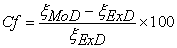(8)

Critical analysis of Figure 13, Figure 14 and Table 6 indicates that the evaluated correction factors are negative of the deviation as shown in equations (7) and (8).

Figure 13, Figure 14 and Table 6 show that the least and highest correction factor (to the model-predicted oil production level) are + 0.75 and + 6.31%, since correction factor is the negative of deviation as shown in equations (7) and (8), Figure 13, Figure 14 and Table 6 indicate that these highlighted correction factors correspond to oil production levels: 1351.851 and 2103.366 MTSB, operating cost: 23666 and 37306 M\$, expected revenue: 24516 and 38156 M\$ as well as current oil price: 18 and 17 \$Bbl respectively.

#### Table 6. Variation of correction factor (to model-predicted oil production level) with operating cost, current oil price and expected revenue

The correction factor took care of the negligence of operational contributions of the prevailing process conditions which actually played vital role during the oil production. The model predicted results deviated from those of the experiment because these contributions were not considered during the model formulation. Introduction of the corresponding values of Cf from equation (8) into the model gives exactly the corresponding experimental values of oil production level.

It is very pertinent to state that the deviation of model predicted results from that of the experiment is just the magnitude of the value. The associated sign preceding the value signifies that the deviation is a deficit (negative sign) or surplus (positive sign).

### 5. Conclusion

Following an evaluation of the trend of oil production level dependence on its operating cost, current oil price and expected revenue over a production period of 10 years (2003-2012), oil production level is very significantly affected by its operating cost and expected revenue at a particular current oil price. The validity of the derived three-factorial model used for the response analysis was rooted in the model core expression ζ - 10-6₰ - 0.1407 = 3 x 10-8 ɤ2 + 0.0488ɤ + 0.0551 ϑ where both sides of the expression are correspondingly approximately equal. The standard error incurred in predicting oil production level for each value of the expected revenue & operating cost considered, as obtained from derived model and regression model were 0.0401and 3.5 x 10-4 & 3.7348 and 3.7544 % respectively. Oil production per unit operating cost as obtained from experiment; derived model and regression model were 0.0556, 0.0552 and 0.0552 MTSB /M\$ respectively. The correlations between oil production and operating cost, current oil price and expected revenue as obtained from experiment; derived model and regression model were all > 0.99. The maximum deviation of the model-predicted oil production level (from experimental results) was less than 7%. This translated into over 93% operational confidence for the derived model as well as over 0.93 reliability response coefficients of oil production dependence to the operating cost, current oil price and expected revenue.

### References

  Mbanugo, C. E., Agunwamba, J. C. and Eke, M. N. (2011). Risk Analysis Applied in Oil Exploration and Production, Nigerian Journal of Technology, 30 (2) 73-79.In article  Newendrop, P. D., and Campbell, J. M. (1971). Expected Value - A Logic for Decisison Making, paper SPE 3327.In article  Macdonald, R. C. and Campbell, J. E. (1983). Evaluation Enhanced Oil Recovery Risk Analysis, paper SPE 11303 presented at the 1983 SPE Hydrocarbon Economics and Evaluation Symposium, Dallas, March 3-4.In article  Startzman, R. A. and Wattenbarger, R. A. (1985).An Improved Computation Procedure for Risk Analysis Problems with unusual Probability Function, paper SPE 13772 presented at the 1985 SPE Hydrocarbon Economics and Evaluation Symposium (Dallas).In article  Davidson, L. B. (2001). Practical Issues in using Risk-Based Decision Analysis, paper SPE 71417 presented at 2001 SPE Annual Technical Conference, New Orleans, L. A, September 30-October 3.In article  Alexander, J. A. and Lohr, J. R. (1998). Risk-Analysis; Lessons Learned, paper SPE 4930 presented at the SPE Annual Technical Conference, New Orleans, L. A, September 27-30.In article  Ikoku, C. U. (1985). Economic Analysis and Investment Decisions, John Wiley & Sons, Inc. New York.In article  Nwoye, C. I. (2008). Data Analytical Memory; C-NIKBRAN.In article